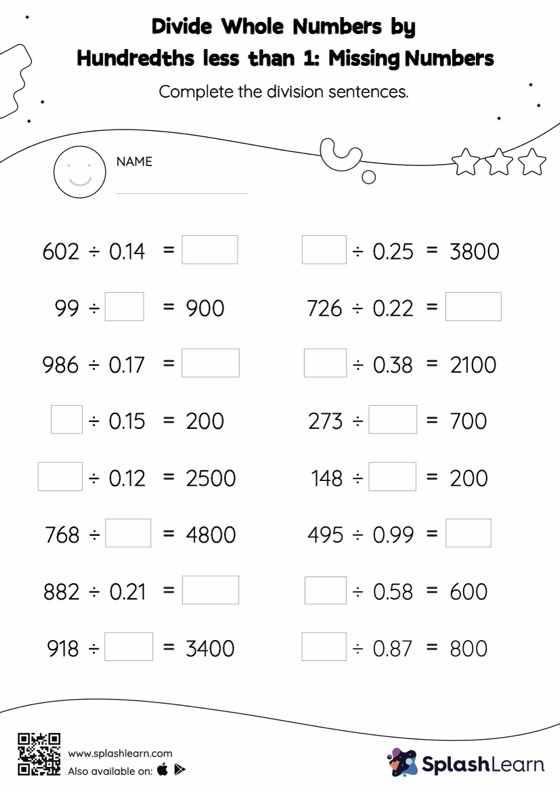# Divide Whole Numbers by Hundredths less than 1 without Remainder: Missing Numbers Worksheet

Home > Divide Whole Numbers by Hundredths less than 1 without Remainder: Missing NumbersWhen dividing a whole number by a decimal, students divide without considering the decimal point and then place the decimal point at the appropriate place in the quotient based on the number of decimal digits in each number. Divide whole numbers by hundredths less than 1 without remainder worksheet requires students to apply this concept to find the missing number. This worksheet is about practicing with the horizontal format in which numbers are written side by side. To develop flexibility with numbers and operations, students need to have enough practice in this format and not just rely on the vertical/column method.